﻿

### Flokki planes and cubic polynomials

#### Abstract

Non-Desarguesian translation planes of order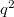$q^2$ are constructed whenever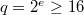$q=2^e\ge 16$ and$e$ isnot divisible by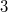$3$. Each plane has kernel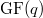$\textnormal{GF}(q)$  andtranslation complement of order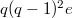$q(q-1)^2 e$, with orbits of lengths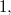$1,$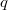$q$ and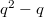$q^2-q$ on the translation line. The planes have elation groups oforder$q$ that producederivable nets, but are not flock planes, semifield planes,  orlifted planes.
The same algebraic tools are used to construct  non-Desarguesiantranslation planes of order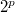$2^p$ for every prime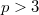$p>3$.

DOI Code: 10.1285/i15900932v29n1supplp211

Keywords:
translation planes

Full Text: PDF